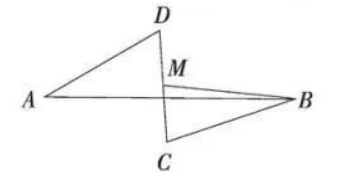$\text{A.}$ 3 $\text{B.}$ 5 $\text{C.}$ 6 $\text{D.}$ 8

D